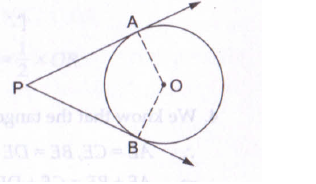# To draw a pair of tangents to a circle, which are inclined to each other at angle of 450 ,`
Question:

To draw a pair of tangents to a circle, which are inclined to each other at angle of 450 , we have to draw the tangents at the end points of those two radii, the angle between which is

(a) 1050
(b) 1350
(c) 1400
(d) 1450

Solution:Suppose PA and PB are two tangents we want to draw which inclined at an angle of 450
We know that the radius and tangent are perperpendular at their point of contact
∵∠OBP = ∠OAP = 900